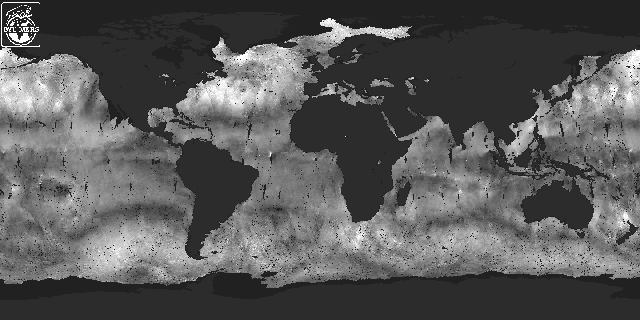Home PageFAQPublicationsDocumentationSoftwareMoviesImage GalleryImage DataData SearchDerived ProductsRelated LinksContact UsSCP file site### NSCAT Probability Distribution

NSCAT makes only indirect measurements of wind. The direct measurement is of the backscattered radar power. This signal power is contaminated by radiometric noise so a separate measurement of the noise power is subtracted from the signal+noise measurement to estimate the backscattered power. Using the radar equation, sigma-0 is computed from the measured signal power. From multiple sigma-0 measurements made at different azimuth angles, the wind is estimated. In wind retrieval, the NSCAT sigma-0 measurements are assumed to have a Gaussian probability distribution with a variance which depends on the mean. Given this distribution model, the maximum-likelihood estimator is formed and optimized to estimate the wind.

Because of the on-board signal processing used by NSCAT, the model of a Gaussian distribution for sigma-0 is only an approximation to the actual distribution. Working from first principles and the design of the NSCAT signal processor we derive the distribution of the NSCAT measurements as a function of the surface sigma-0, the signal to noise ratio and the cell number. The resulting hypergeometric distribution is skewed relative to the traditional Gaussian model. This PDF has been derived by Paul E. Johnson, Uncertainties in Oceanic Microwave Remote Sensing: the Radar Footprint, the Wind-Backscatter Relationship, and the Measurement Probability Density Function, Ph.D. Dissertation, Brigham Young University, Provo, Utah, 1999. (A related paper is P.J. Johnson and D.G. Long, "The Probability Density of Spectral Estimates Based on Modified Periodogram Averages," IEEE Transactions on Signal Processing, Vol. 47, No. 5, pp. 1255-1261, 1999.)

The observed NSCAT probability distribution (pdf) will be the combination (convolution) of the pdf described above and the pdf of the sigma-0 of the surface. The latter will be a function of the pdf of the near-surface wind field and the geophysical model function.

From wavetank and lake experiments there is strong evidence that sigma-0 falls off rapidly at low winds. While this effect is clear in such data, it is not as clear in spaceborne data where the radar footprint covers 25² km or more. However, if sigma-0 does drop to low values at low wind speeds, the probability of seeing negative sigma-0 measurements (which occur because of noise in the power measurements) increases. As evidence that negative sigma-0 measurements are correlated with low wind speeds consider the following figures. Figure 1 shows the spatial distribution of negative sigma-0 measurements over a three day period. Figure 2 shows a map of 3 days of NSCAT-derived wind speeds. Figure 3 shows shows a map of the standard deviation of NSCAT-derived wind speeds over the three day period. The later image is useful in evaluting the temporal variation over the three day period.

Figure 1: Map of the count of the number of negative sigma-0 measurements in 3 days of NSCAT data. This is a shunk down version of the original image (58 K) which is a 1/4 deg by 1/4 deg map. In this image, the number of measurements in each grid element are indicated by a gray value with white as the most (thresholded to 5).Figure 2: Map of the average NSCAT-derived wind speed over a three day period. This is a shunk down version of the original image (605 K) which is a 1/4 deg by 1/4 deg map. Whiter values indicate higher wind speeds with pure white 18 m/s and higher.Figure 3: Map of the average NSCAT-derived wind speed over a three day period. This is a shunk down version of the original image (608 K) which is a 1/4 deg by 1/4 deg map. Whiter values indicate higher wind speeds with pure white 18 m/s and higher.Figure 4: Map of the count of the number of sigma-0 smaller (but positive) than the square of root of Gamma in the quadratic Kpc equation in 3 days of NSCAT data. This is a shunk down version of the original image (58 K) which is a 1/4 deg by 1/4 deg map. In this image, the number of measurements in each grid element are indicated by a gray value with white as the most (thresholded to 5).Figure 5: Map of the weekly NCEP analyzed sea surface temperture (SST). This is a shunk down version of the original image (136 K) which is a 1/4 deg by 1/4 deg map. In this image, the temperature is represented as a gray value from -10 to 40 deg C.The images presented above are for JDs 8-10 in 1997. Full size images for JDs 19-21 are available below.
Spatial distribution negative sigma-0 image (55 K)
Wind speed image (619 K)
Wind speed standard deviation image (621 K)
Spatial distribution of low sigma-0 values (58 K)
SST map (135 K)